# Solution assignment 07 Fractional functions and graphs

### Assignment 7

Given the function: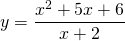Find:
the vertical asymptote;
the horizontal asymptote;
the intersection point with the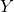-as if it exists;
the intersection point with the-as if it exists.

Based on these results sketch the result in the figure.

### Solution

The line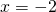seems to be an asymptote. However, when we substitute this value in the formula we get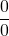. That the numerator yields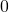formeans that the numerator contains the factor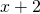. Indeed, the numerator can be factorized: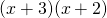. Now the function can be rewritten: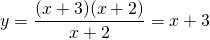if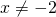.

We do not need to do complicated calculations any more. There also no asymptotes. The graph of the function is equal to the graph of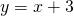, excluded the point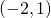. The graph approaches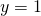ifapproaches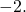The graph in the figure is valid, exluded the point.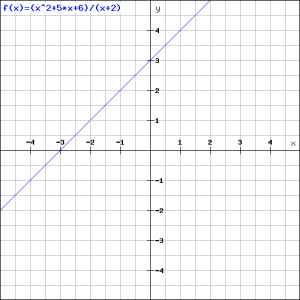0